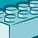# QlikView App Dev

Discussion Board for collaboration related to QlikView App Development.

cancel
Showing results for
Did you mean:Contributor III

## Compare and filter values in two columns based on their difference i

Hi,

I have been struggling to add a filter or flag for comparing Sum1 and Sum2? I would like to be able to choose which product Ids have Sum2-Sum1 = 0 and which ones not equal to 0. The ideal soultion would be a flag as filter having both 0 and 'not zero', so if I click 0 I will have only rows with Ids 1 and 4 displayed. Any idea? Thanks in advance.

IdSum1Sum2

1

500500
2750400
320090
4800800
1 Solution

Accepted SolutionsChampion III

But let's say they aren't fields in your data model, but calculations in a chart. Say your data has an ID, and fields One and Two, and you're showing a chart of ID, sum(One), and sum(Two). You can make a list box with this expression:

aggr(if(sum(Two)-sum(One)=0,0,'Not 0'),ID)

See attached.

5 RepliesChampion III

If Sum1 and Sum2 are actual fields in your table, not just in a chart, then in script:

if(Sum2=Sum1,0,'Not 0') as [Difference Flag],Champion III

But let's say they aren't fields in your data model, but calculations in a chart. Say your data has an ID, and fields One and Two, and you're showing a chart of ID, sum(One), and sum(Two). You can make a list box with this expression:

aggr(if(sum(Two)-sum(One)=0,0,'Not 0'),ID)

See attached.Contributor III
Author

Hello John,

This solution seems to be exactly what I need, however, I got an empty List Box. I have this kind of expression:

=aggr(if(Sum(2)-SUM(1)/1000=0,0,'Not 0'),ID)

I got no error message in the expression box. I had to divide SUM(1) by 100 to get the same units as SUM2.Champion III

What's your actual expression? Might be as simple as a syntax error.Contributor III
Author

You were right Mr. John, I had to replace 'ID' with the new dimension name that I'm using. Now it works!

Thanks a million. Cheers!